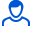Content: teorver_5575.doc (28.00 KB)

Positive responses: 0
Negative responses: 0

Refunds: 05575. x̅=6, n=900, σ=32. Determine the confidence interval for estimating with reliability γ=0.989 the unknown mathematical expectation a of the normally distributed feature X of the general population, if the sample mean x̅в=6, the sample size n=900 and the general standard deviation σ=32 are known.
Detailed solution. Decorated in Microsoft Word 2003 (Quest decided to use the formula editor)
No feedback yet